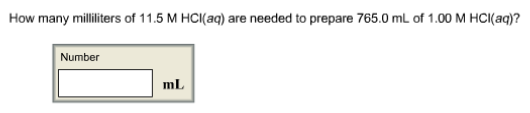# Problem: How many milliliters of 11.5 M HCI(aq) are needed to prepare 765.0 mL of 1.00 M HCl(aq)?

###### FREE Expert Solution

Using the dilution equation:

95% (381 ratings)###### Problem Details

How many milliliters of 11.5 M HCI(aq) are needed to prepare 765.0 mL of 1.00 M HCl(aq)?